# Stacked Graph

ConceptDraw DIAGRAM is a powerful software for drawing professional looking Stacked Graph thanks to the Divided Bar Diagrams solution from the Graphs and Charts area of ConceptDraw Solution Park.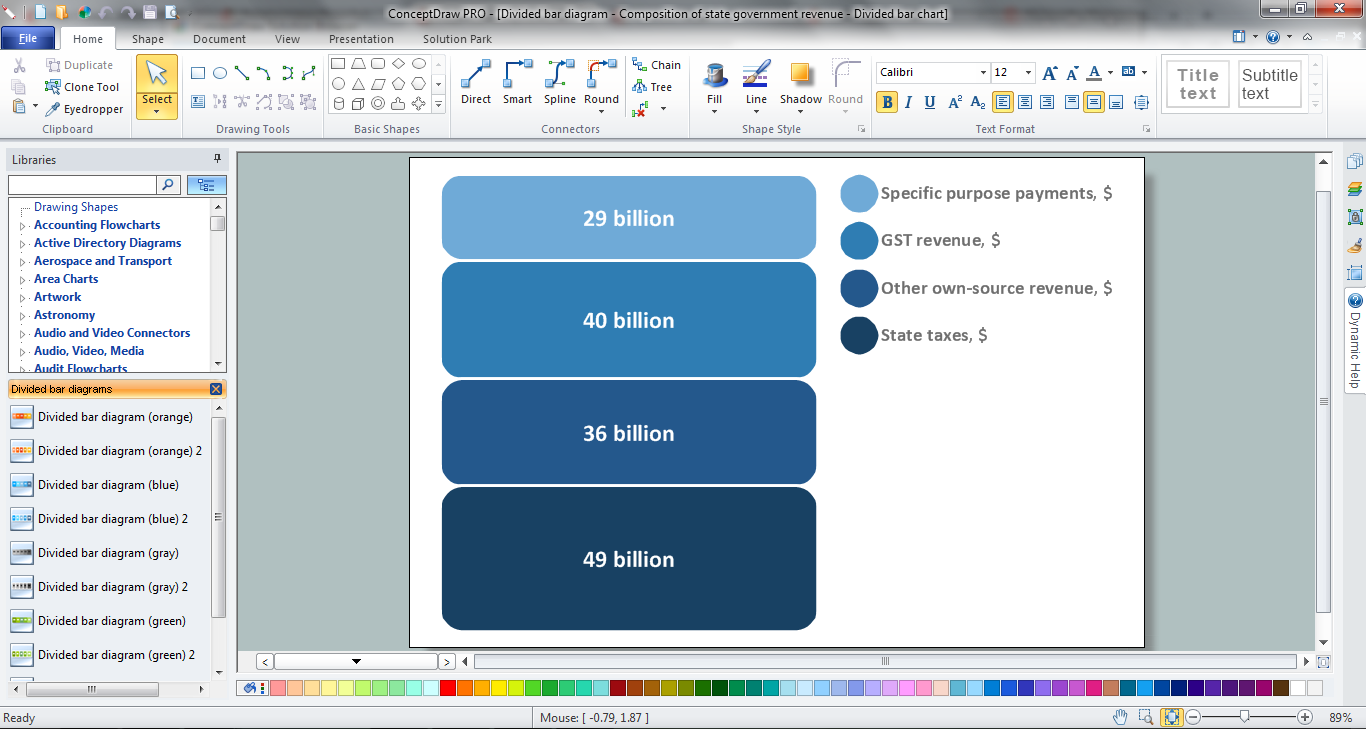Example 1. Stacked Graph in ConceptDraw DIAGRAM /p>

Divided Bar Diagrams solution extends ConceptDraw DIAGRAM (or later) with templates, samples and Divided Bar Diagrams library with design elements for drawing the Stacked Graph of any complexity.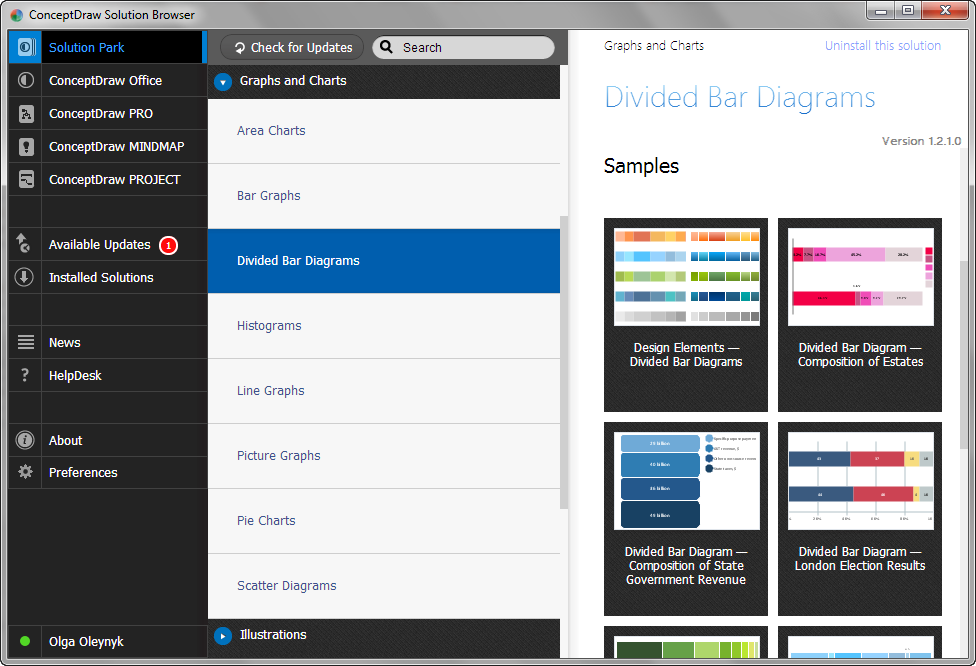Example 2. Divided Bar Diagrams Solution in ConceptDraw STORE

Divided Bar Diagrams and Stacked Graph examples, templates and samples are represented in ConceptDraw STORE. They are available for viewing and editing.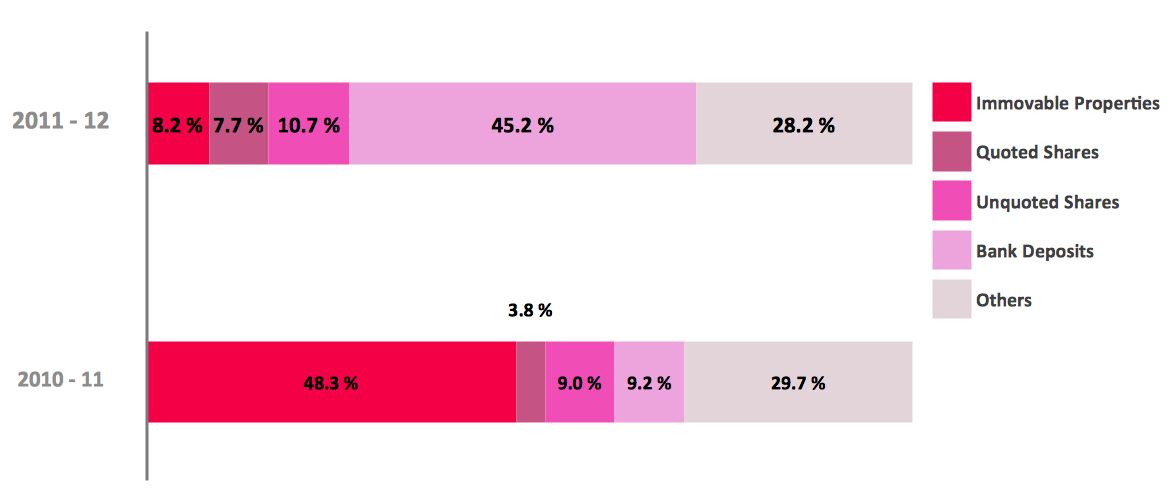Example 3. Stacked Graph - Composition of estates

This sample was created in ConceptDraw DIAGRAM using the Divided Bar Diagrams solution from the Graphs and Charts area of ConceptDraw Solution Park. It shows a stacked graph of composition of estates. An experienced user spent 10 minutes creating this sample.

The Stacked Graph and Divided Bar Diagrams produced with ConceptDraw DIAGRAM is vector graphic documents and is available for reviewing, modifying, converting to a variety of formats (image, HTML, PDF file, MS PowerPoint Presentation, Adobe Flash or MS Visio), printing and send via e-mail in one moment.

Related Solution:

### Bar Graph

You need to draw the Bar Graph? The automated tool can be useful for you. ConceptDraw DIAGRAM v12 diagramming and vector drawing software extended with Bar Graphs solution from Graphs and Charts area of ConceptDraw Solution Park will help you create any Bar Graph.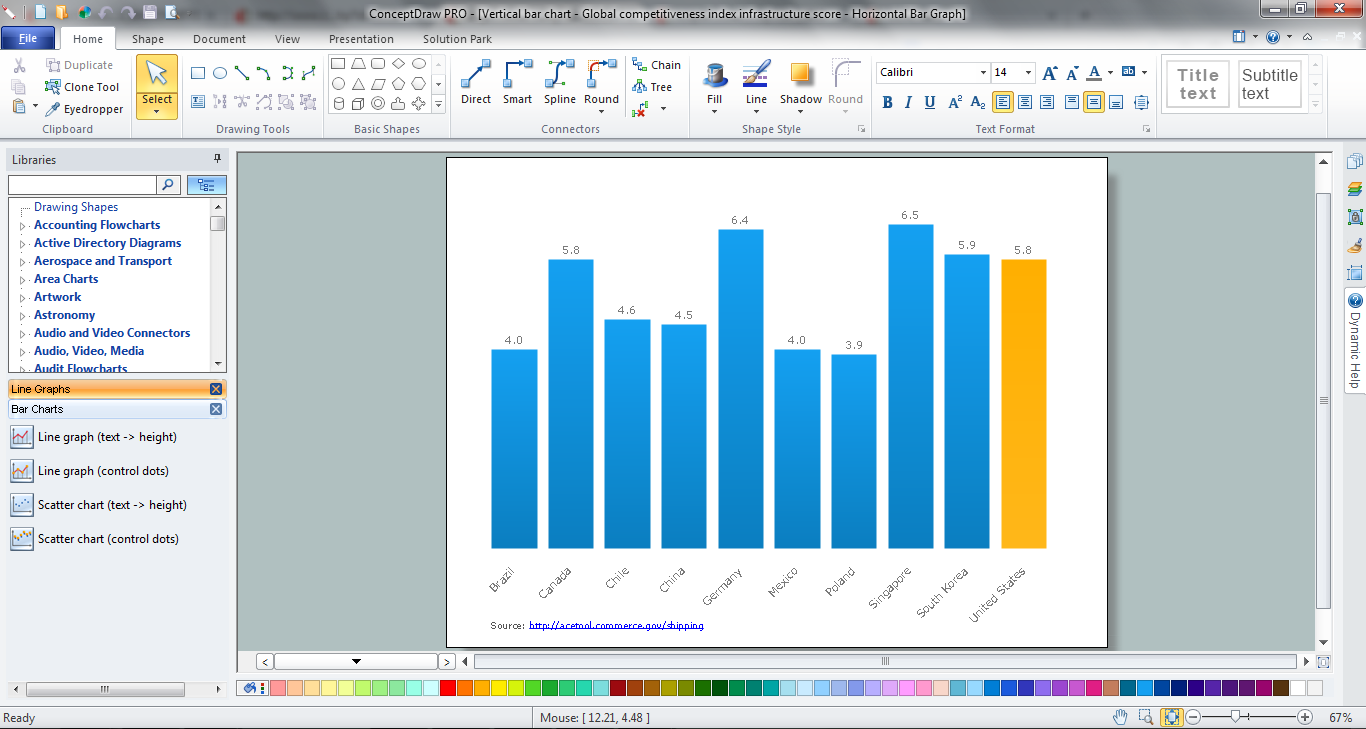Picture: Bar Graph
Related Solution:

### Bar Chart

ConceptDraw DIAGRAM v12 extended with Bar Graphs solution from Graphs and Charts area of ConceptDraw Solution Park is ideal software for quick and simple drawing bar chart of any complexity graph.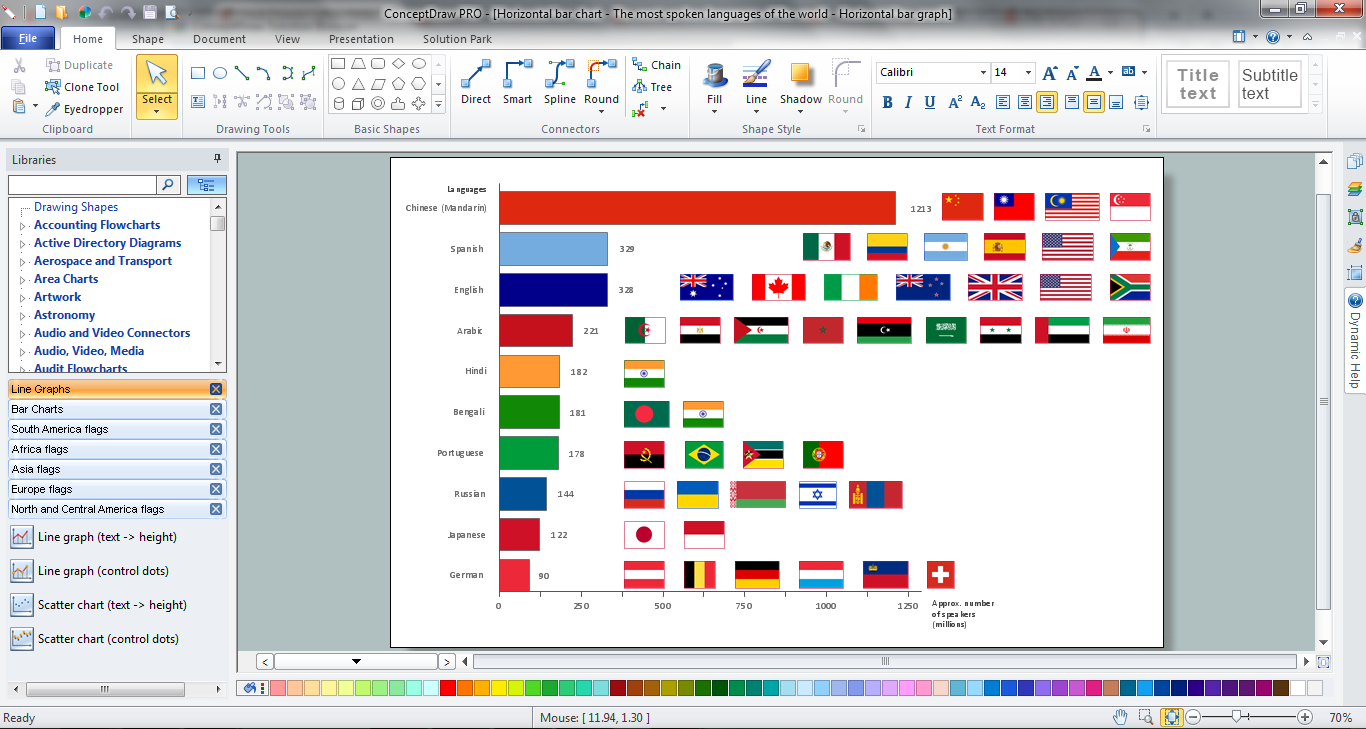Picture: Bar Chart
Related Solution:

### Bar Diagram Math

ConceptDraw DIAGRAM v12 extended with Divided Bar Diagrams solution from Graphs and Charts area of ConceptDraw Solution Park is the best software for quick and simple drawing the Divided Bar Diagrams and Bar Diagram Math.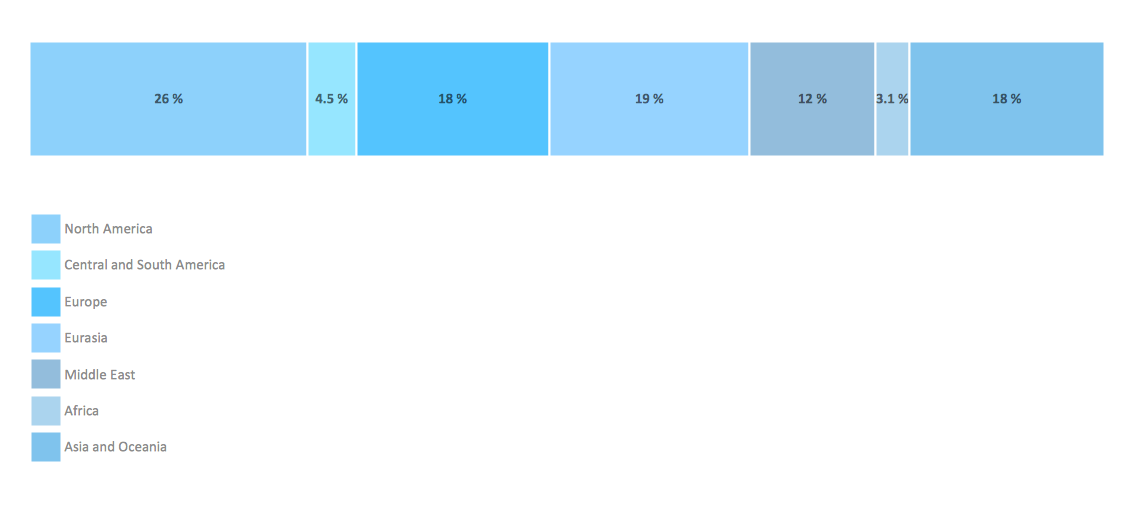Picture: Bar Diagram Math
Related Solution: